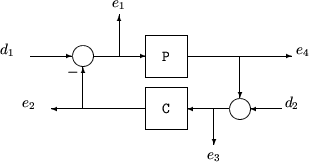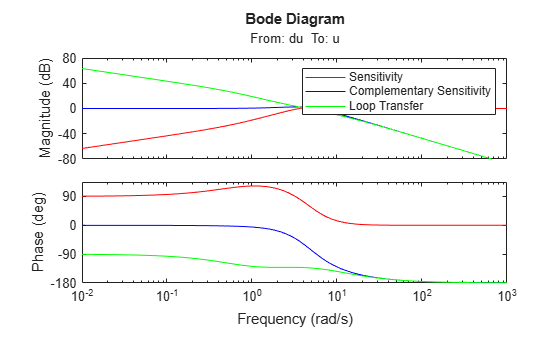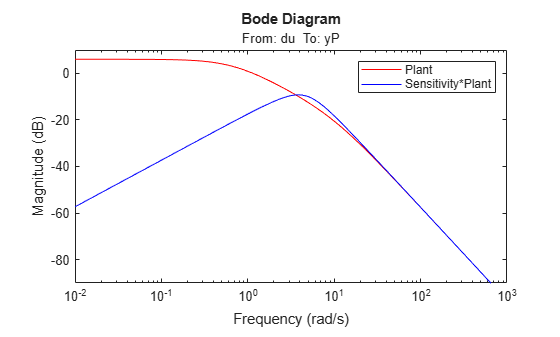# loopsens

Sensitivity functions of plant-controller feedback loop

## Syntax

```loops = loopsens(P,C)
```

## Description

`loops = loopsens(P,C)` creates a `struct`, `loops`, whose fields contain the multivariable sensitivity, complementary and open-loop transfer functions. The closed-loop system consists of the controller `C` in negative feedback with the plant `P`. `C` should only be the compensator in the feedback path, not any reference channels, if it is a 2-Dof controller as seen in the figure below. The plant and compensator `P` and `C` can be constant matrices, `double, lti` objects, `frd/ss/tf/zpk`, or uncertain objects `umat/ufrd/uss`.The `loops` returned variable is a structure with fields:

Field

Description

`Poles`

Closed-loop poles. `NaN` for `frd/ufrd` objects

`Stable`

1 if nominal closed loop is stable, 0 otherwise. `NaN` for `frd/ufrd` objects

`Si`

Input-to-plant sensitivity function

`Ti`

Input-to-plant complementary sensitivity function

`Li`

Input-to-plant loop transfer function

`So`

Output-to-plant sensitivity function

`To`

Output-to-plant complementary sensitivity function

`Lo`

Output-to-plant loop transfer function

`PSi`

Plant times input-to-plant sensitivity function

`CSo`

Compensator times output-to-plant sensitivity function

The multivariable closed-loop interconnection structure, shown below, defines the input/output sensitivity, complementary sensitivity, and loop transfer functions.The following table gives the values of the input and output sensitivity functions for this control structure.

Description

Equation

Input sensitivity Si (closed-loop transfer function from d1 to e1)

Si = (I + CP)–1

Input complementary sensitivity Ti (closed-loop transfer function from d1 to e2)

Ti = CP(I + CP)–1

Output sensitivity So (closed-loop transfer function from d2 to e2)

So = (I + PC)–1

Output complementary sensitivity To (closed-loop transfer function from d2 to e4)

To = PC(I + PC)–1

Input loop transfer function Li

Li = CP

Output loop transfer function Lo

Lo = PC

## Examples

collapse all

Consider PI controller for a dominantly 1st-order plant, with the closed-loop bandwidth of 2.5 rads/sec. Since the problem is SISO, all gains are the same at input and output.

```gamma = 2; tau = 1.5; taufast = 0.1; P = tf(gamma,[tau 1])*tf(1,[taufast 1]); tauclp = 0.4; xiclp = 0.8; wnclp = 1/(tauclp*xiclp); KP = (2*xiclp*wnclp*tau - 1)/gamma; KI = wnclp^2*tau/gamma; C = tf([KP KI],[1 0]);```

Form the closed-loop (and open-loop) systems with `loopsens`, and plot Bode plots using the gains at the plant input.

```loops = loopsens(P,C); bode(loops.Si,'r',loops.Ti,'b',loops.Li,'g')```Finally, compare the open-loop plant gain to the closed-loop value of `PSi`.

`bodemag(P,'r',loops.PSi,'b')`Consider an integral controller for a constant-gain, 2-input, 2-output plant. For purposes of illustration, the controller is designed via inversion, with different bandwidths in each rotated channel.

```P = ss([2 3;-1 1]); BW = diag([2 5]); [U,S,V] = svd(P.d); % get SVD of Plant Gain Csvd = V*inv(S)*BW*tf(1,[1 0])*U'; % inversion based on SVD loops = loopsens(P,Csvd); bode(loops.So,'g',loops.To,'r.',logspace(-1,3,120)) title('Output Sensitivity (green), Output Complementary Sensitivity (red)');```